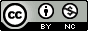## Monday, November 9, 2009

### QQ plots of p-values in R using ggplot2

Way back will wrote on this topic.  See his previous post for Stata code for doing this.  Unfortunately the R package that was used to create QQ-plots here has been removed from CRAN, so I wrote my own using ggplot2 and some code I received from Daniel Shriner at NHGRI.

Of course you can use R's built-in qqplot() function, but I could never figure out a way to add the diagonal using base graphics.  To get the function I wrote to make qqplots, copy and paste this into your R session:

qq = function(pvector, title="Quantile-quantile plot of p-values", spartan=F) {
# Thanks to Daniel Shriner at NHGRI for providing this code for creating expected and observed values
o = -log10(sort(pvector,decreasing=F))
e = -log10( 1:length(o)/length(o) )
# you could use base graphics
#plot(e,o,pch=19,cex=0.25, xlab=expression(Expected~~-log(italic(p))), ylab=expression(Observed~~-log(italic(p))),xlim=c(0,max(e)),ylim=c(0,max(e)))
#lines(e,e,col="red")
#You'll need ggplot2 installed to do the rest
plot=qplot(e,o, xlim=c(0,max(e)), ylim=c(0,max(o))) + stat_abline(intercept=0,slope=1, col="red")
plot=plot+opts(title=title)
plot=plot+scale_x_continuous(name=expression(Expected~~-log(italic(p))))
plot=plot+scale_y_continuous(name=expression(Observed~~-log(italic(p))))
if (spartan) plot=plot+opts(panel.background=theme_rect(col="grey50"), panel.grid.minor=theme_blank())
plot
}

Also, make sure you have ggplot2 installed:

install.packages("ggplot2")

If you already have it installed, load it:

library(ggplot2)

The function takes a vector of p-values, optionally a title, and a third option to make the plot black and white.  You can check the default arguments to the function by typing args(qq).

qq(data\$Pvals, title="My Quantile-Quantile Plot")

You can also make the plot more "Spartan" by removing the title (setting it to NULL) and by making the color scheme black on white:

qq(data\$Pvals, title=NULL, spartan=TRUE)

Stay tuned - I'm also putting together a way to import your PLINK output files and make better Manhattan plots using R and ggplot2 than you ever could with Haploview.

Update Tuesday, November 10, 2009: A big tip of the hat to the anonymous commenter who pointed out that this can easily be done in the base graphics package, as well as adding confidence intervals.  As always, we welcome your comments if you know of a better or easier way to do anything mentioned here!

#### 6 comments:

1.Diagonal in base graphics: do you mean qqline or abline(c(1,1))?

2.abline(0,1,col="red") is how to add the diagonal in the base package.

In the base package you can also add confidence intervals fairly easily. These assume independence, so are conservative in the presence of LD.

## obs <- readfile; p-values only
## read in your p-values,
## here I generated some
obs <- -log(c(runif(99000,0,1),rbeta(1000,0.5,1)),10)
N <- length(obs) ## number of p-values

## create the null distribution
## (-log10 of the uniform)
null <- -log(1:N/N,10)
MAX <- max(c(obs,null))

## create the confidence intervals
c95 <- rep(0,N)
c05 <- rep(0,N)

## the jth order statistic from a
## uniform(0,1) sample
## has a beta(j,n-j+1) distribution
## (Casella & Berger, 2002,
## 2nd edition, pg 230, Duxbury)

for(i in 1:N){
c95[i] <- qbeta(0.95,i,N-i+1)
c05[i] <- qbeta(0.05,i,N-i+1)
}

## plot the two confidence lines
plot(null, -log(c95,10), ylim=c(0,MAX), xlim=c(0,MAX), type="l",
axes=FALSE, xlab="", ylab="")
par(new=T)
plot(null, -log(c05,10), ylim=c(0,MAX), xlim=c(0,MAX), type="l",
axes=FALSE, xlab="", ylab="")
## add the diagonal
abline(0,1,col="red")
par(new=T)

## add the qqplot
qqplot(null,obs, ylim=c(0,MAX),xlim=c(0,MAX), main="Yay! It's a QQPlot")

3.if you have a "XXX.txt" file with single column of p-values, what would be the correct first line of the script? I tried obs <- read.table("XXX.txt"), but when I run the entire script, why it gave me the error:

> MAX <- max(c(obs,null))
Error in max(c(obs, null)) : invalid 'type' (list) of argument
>
>
> ## create the confidence intervals
> c95 <- rep(0,N)
> c05 <- rep(0,N)
>
> ## the jth order statistic from a
> ## uniform(0,1) sample
> ## has a beta(j,n-j+1) distribution
> ## (Casella & Berger, 2002,
> ## 2nd edition, pg 230, Duxbury)
>
> for(i in 1:N){
+ c95[i] <- qbeta(0.95,i,N-i+1)
+ c05[i] <- qbeta(0.05,i,N-i+1)
+ }
>
> ## plot the two confidence lines
> plot(null, -log(c95,10), ylim=c(0,MAX), xlim=c(0,MAX), type="l",
+ axes=FALSE, xlab="", ylab="")
> par(new=T)
> plot(null, -log(c05,10), ylim=c(0,MAX), xlim=c(0,MAX), type="l",
+ axes=FALSE, xlab="", ylab="")
> ## add the diagonal
> abline(0,1,col="red")
> par(new=T)
>
> ## add the qqplot
> qqplot(null,obs, ylim=c(0,MAX),xlim=c(0,MAX), main="Yay! It's a QQPlot")
Error in order(list(V1 = c(3.78489141894691, 3.63189914829065, 3.52331325705436, :
unimplemented type 'list' in 'orderVector1'

4.How Can I calculate the Genomic Inflation Factor by R? It is very important to know the inflation factor in GWAS expecially when a QQplot is shown.
Thanks!

5.This is a very nice script! May I ask you how to calculate and add the 95% 'concentration bands' as shade in the Q-Q plot? This should be formed by calculating,for each order statistic,the 2.5th and 97.5th centiles of the distribution of the order statistic under random sampling and the null hypothesis as indicated by WTCCC in Nature.2007,477:661-678.
Thanks very much!

Dan
simpledandan@gmail.com

6.should the expectations not be
e = -log10( (1:length(o)-0.5)/length(o) )
instead of
e = -log10( 1:length(o)/length(o) )
?

Note: Only a member of this blog may post a comment.Getting Genetics Done by Stephen Turner is licensed under a Creative Commons Attribution-NonCommercial 3.0 Unported License.